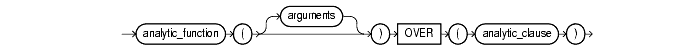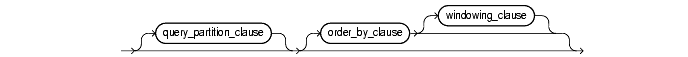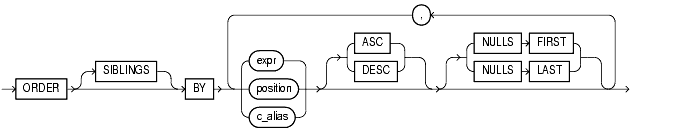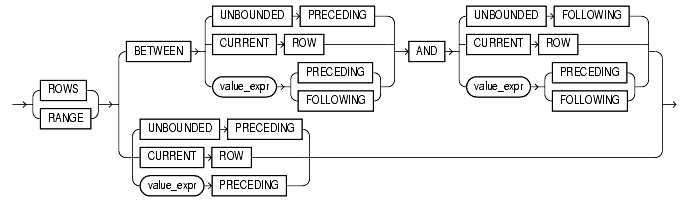Functions, 2 of 166

## SQL Functions

SQL functions are built into Oracle and are available for use in various appropriate SQL statements. Do not confuse SQL functions with user functions written in PL/SQL.

If you call a SQL function with an argument of a datatype other than the datatype expected by the SQL function, Oracle implicitly converts the argument to the expected datatype before performing the SQL function. If you call a SQL function with a null argument, the SQL function automatically returns null. The only SQL functions that do not necessarily follow this behavior are `CONCAT`, `NVL`, and `REPLACE`.

In the syntax diagrams for SQL functions, arguments are indicated by their datatypes. When the parameter "function" appears in SQL syntax, replace it with one of the functions described in this section. Functions are grouped by the datatypes of their arguments and their return values.

 Note: When you apply SQL functions to LOB columns, Oracle creates temporary LOBs during SQL and PL/SQL processing. You should ensure that temporary tablespace quota is sufficient for storing these temporary LOBs for your application.

 See Also: "User-Defined Functions" for information on user functions Oracle Text Reference for information on functions used with Oracle Text "Data Conversion" for implicit conversion of datatypes

The general syntax is as follows:

function::=Text description of `function`

single_row_function::=Text description of `single_row_function`

The sections that follow list the built-in SQL functions in each of the groups illustrated above except user-defined functions. All of the built-in SQL functions are then described in alphabetical order. User-defined functions are described at the end of this chapter.

### Single-Row Functions

Single-row functions return a single result row for every row of a queried table or view. These functions can appear in select lists, `WHERE` clauses, `START` `WITH` and `CONNECT` `BY` clauses, and `HAVING` clauses.

#### Number Functions

Number functions accept numeric input and return numeric values. Most of these functions return values that are accurate to 38 decimal digits. The transcendental functions `COS`, `COSH`, `EXP`, `LN`, `LOG`, `SIN`, `SINH`, `SQRT`, `TAN`, and `TANH` are accurate to 36 decimal digits. The transcendental functions `ACOS`, `ASIN`, `ATAN`, and `ATAN2` are accurate to 30 decimal digits. The number functions are:

#### Character Functions Returning Character Values

Character functions that return character values return values of the same datatype as the input argument.

• Functions that return `CHAR` values are limited in length to 2000 bytes.

• Functions that return `VARCHAR2` values are limited in length to 4000 bytes.

For both of these types of functions, if the length of the return value exceeds the limit, Oracle truncates it and returns the result without an error message.

• Functions that return `CLOB` values are limited to 4 GB.

For `CLOB` functions, if the length of the return values exceeds the limit, Oracle raises an error and returns no data.

The character functions that return character values are:

#### Character Functions Returning Number Values

Character functions that return number values can take as their argument any character datatype.

The character functions that return number values are:

#### Datetime Functions

Date functions operate on values of the `DATE` datatype. All date functions return a datetime or interval value of `DATE` datatype, except the `MONTHS_BETWEEN` function, which returns a number. The date functions are:

#### Conversion Functions

Conversion functions convert a value from one datatype to another. Generally, the form of the function names follows the convention `datatype` `TO` `datatype`. The first datatype is the input datatype. The second datatype is the output datatype. The SQL conversion functions are:

#### Miscellaneous Single-Row Functions

The following single-row functions do not fall into any of the other single-row function categories.

### Aggregate Functions

Aggregate functions return a single result row based on groups of rows, rather than on single rows. Aggregate functions can appear in select lists and in `ORDER` `BY` and `HAVING` clauses. They are commonly used with the `GROUP` `BY` clause in a `SELECT` statement, where Oracle divides the rows of a queried table or view into groups. In a query containing a `GROUP` `BY` clause, the elements of the select list can be aggregate functions, `GROUP` `BY` expressions, constants, or expressions involving one of these. Oracle applies the aggregate functions to each group of rows and returns a single result row for each group.

If you omit the `GROUP` `BY` clause, Oracle applies aggregate functions in the select list to all the rows in the queried table or view. You use aggregate functions in the `HAVING` clause to eliminate groups from the output based on the results of the aggregate functions, rather than on the values of the individual rows of the queried table or view.

 See Also: "GROUP BY Examples" and the "HAVING Clause" for more information on the `GROUP` `BY` clause and `HAVING` clauses in queries and subqueries

Many (but not all) aggregate functions that take a single argument accept these clauses:

• `DISTINCT` causes an aggregate function to consider only distinct values of the argument expression.

• `ALL` causes an aggregate function to consider all values, including all duplicates.

For example, the `DISTINCT` average of 1, 1, 1, and 3 is 2. The `ALL` average is 1.5. If you specify neither, the default is `ALL`.

All aggregate functions except `COUNT`(*) and `GROUPING` ignore nulls. You can use the `NVL` function in the argument to an aggregate function to substitute a value for a null. `COUNT` never returns null, but returns either a number or zero. For all the remaining aggregate functions, if the data set contains no rows, or contains only rows with nulls as arguments to the aggregate function, then the function returns null.

You can nest aggregate functions. For example, the following example calculates the average of the maximum salaries of all the departments in the `scott` schema:

```SELECT AVG(MAX(salary)) FROM employees GROUP BY department_id;

AVG(MAX(SALARY))
----------------
10925

```

This calculation evaluates the inner aggregate (`MAX`(`sal`)) for each group defined by the `GROUP` `BY` clause (`deptno`), and aggregates the results again.

The aggregate functions are:

### Analytic Functions

Analytic functions compute an aggregate value based on a group of rows. They differ from aggregate functions in that they return multiple rows for each group. The group of rows is called a window and is defined by the analytic clause. For each row, a "sliding" window of rows is defined. The window determines the range of rows used to perform the calculations for the "current row". Window sizes can be based on either a physical number of rows or a logical interval such as time.

Analytic functions are the last set of operations performed in a query except for the final `ORDER` `BY` clause. All joins and all `WHERE`, `GROUP` `BY`, and `HAVING` clauses are completed before the analytic functions are processed. Therefore, analytic functions can appear only in the select list or `ORDER` `BY` clause.

Analytic functions are commonly used to compute cumulative, moving, centered, and reporting aggregates.

analytic_function::=Text description of `analytic_function`

analytic_clause::=Text description of `analytic_clause`Text description of `query_partition_clause`Text description of `order_by_clause`Text description of `windowing_clause`

The keywords and parameters of this syntax are:

#### analytic_function

Specify the name of an analytic function (see the listing of analytic functions following this discussion of keywords and parameters).

#### arguments

Analytic functions take 0 to 3 arguments.

#### analytic_clause

Use `OVER` `analytic_clause` clause to indicate that the function operates on a query result set. That is, it is computed after the `FROM`, `WHERE`, `GROUP` `BY`, and `HAVING` clauses. You can specify analytic functions with this clause in the select list or `ORDER` `BY` clause. To filter the results of a query based on an analytic function, nest these functions within the parent query, and then filter the results of the nested subquery.

 Notes: You cannot specify any analytic function in any part of the analytic_clause. That is, you cannot nest analytic functions. However, you can specify an analytic function in a subquery and compute another analytic function over it. You can specify `OVER` analytic_clause with user-defined analytic functions as well as built-in analytic functions. See CREATE FUNCTION.
##### order_by_clause

Use the order_by_clause to specify how data is ordered within a partition. For all analytic functions except `PERCENTILE_CONT` and `PERCENTILE_DISC` (which take only a single key), you can order the values in a partition on multiple keys, each defined by a value_expr and each qualified by an ordering sequence.

Within each function, you can specify multiple ordering expressions. Doing so is especially useful when using functions that rank values, because the second expression can resolve ties between identical values for the first expression.

 Note: Whenever the order_by_clause results in identical values for multiple rows, the function returns the same result for each of those rows. Please refer to the analytic example for SUM for an illustration of this behavior.

Restriction: When used in an analytic function, the order_by_clause must take an expression (expr). The `SIBLINGS` keyword is not valid (it is relevant only in hierarchical queries). Position (position) and column aliases (c_alias) are invalid. Otherwise this order_by_clause is the same as that used to order the overall query or subquery.

##### ASC | DESC

Specify the ordering sequence (ascending or descending). `ASC` is the default.

##### NULLS FIRST | NULLS LAST

Specify whether returned rows containing null values should appear first or last in the ordering sequence.

`NULLS` `LAST` is the default for ascending order, and `NULLS` `FIRST` is the default for descending order.

 Note: Analytic functions always operate on rows in the order specified in the order_by_clause of the function. However, the order_by_clause of the function does not guarantee the order of the result. Use the order_by_clause of the query to guarantee the final result ordering.

##### windowing_clause

Some analytic functions allow the `windowing_clause`. Table 6-8 lists the analytic functions. The functions that allow the `windowing_clause` are followed by an asterisk.

##### ROWS | RANGE

These keywords define for each row a "window" (a physical or logical set of rows) used for calculating the function result. The function is then applied to all the rows in the window. The window "slides" through the query result set or partition from top to bottom.

• `ROWS` specifies the window in physical units (rows).

• `RANGE` specifies the window as a logical offset.

You cannot specify this clause unless you have specified the order_by_clause.

 Note: The value returned by an analytic function with a logical offset is always deterministic. However, the value returned by an analytic function with a physical offset may produce nondeterministic results unless the ordering expression results in a unique ordering. You may have to specify multiple columns in the order_by_clause to achieve this unique ordering.
##### BETWEEN ... AND

Use the `BETWEEN` ... `AND` clause to specify a start point and end point for the window. The first expression (before `AND`) defines the start point and the second expression (after `AND`) defines the end point.

If you omit `BETWEEN` and specify only one end point, Oracle considers it the start point, and the end point defaults to the current row.

##### UNBOUNDED PRECEDING

Specify `UNBOUNDED` `PRECEDING` to indicate that the window starts at the first row of the partition. This is the start point specification and cannot be used as an end point specification.

##### UNBOUNDED FOLLOWING

Specify `UNBOUNDED` `FOLLOWING` to indicate that the window ends at the last row of the partition. This is the end point specification and cannot be used as a start point specification.

##### CURRENT ROW

As a start point, `CURRENT` `ROW` specifies that the window begins at the current row or value (depending on whether you have specified `ROW` or `RANGE`, respectively). In this case the end point cannot be value_expr `PRECEDING`.

As an end point, `CURRENT` `ROW` specifies that the window ends at the current row or value (depending on whether you have specified `ROW` or `RANGE`, respectively). In this case the start point cannot be value_expr `FOLLOWING`.

##### value_expr PRECEDING or value_expr FOLLOWING

For `RANGE` or `ROW`:

• If value_expr `FOLLOWING` is the start point, then the end point must be value_expr `FOLLOWING`.

• If value_expr `PRECEDING` is the end point, then the start point must be value_expr `PRECEDING`.

If you are defining a logical window defined by an interval of time in numeric format, you may need to use conversion functions.

 See Also: NUMTOYMINTERVAL and NUMTODSINTERVAL for information on converting numeric times into intervals

If you specified `ROWS`:

• value_expr is a physical offset. It must be a constant or expression and must evaluate to a positive numeric value.

• If value_expr is part of the start point, it must evaluate to a row before the end point.

If you specified `RANGE`:

• value_expr is a logical offset. It must be a constant or expression that evaluates to a positive numeric value or an interval literal.

• You can specify only one expression in the order_by_clause

• If value_expr evaluates to a numeric value, the `ORDER` `BY` `expr` must be a `NUMBER` or `DATE` datatype.

• If value_expr evaluates to an interval value, the `ORDER` `BY` `expr` must be a `DATE` datatype.

If you omit the windowing_clause entirely, the default is `RANGE` `BETWEEN` `UNBOUNDED` `PRECEDING` `AND` `CURRENT` `ROW`.

Analytic functions are commonly used in data warehousing environments. The analytic functions listed in Table 6-8. Functions followed by an asterisk allow the full syntax, including the `windowing_clause`.

##### Table 6-8 Analytic Functions
 AVG` *` CORR` *` COVAR_POP` *` COVAR_SAMP` *` COUNT` *` FIRST_VALUE` *` LAST_VALUE` *` MAX` *` MIN` *` REGR_ (linear regression) functions` *` STDDEV` *` STDDEV_POP` *` STDDEV_SAMP` *` SUM` *` VAR_POP` *` VAR_SAMP` *` VARIANCE` *`